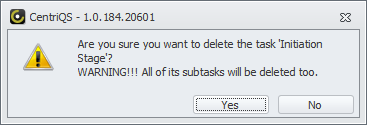You can break tasks into multiple levels of subtasks.To create a subtask

• Click the required task, and then click Add Sub Task on the ribbon, in the Data group.Note The Parent box in the Task dialog box shows what task the given subtask belongs to.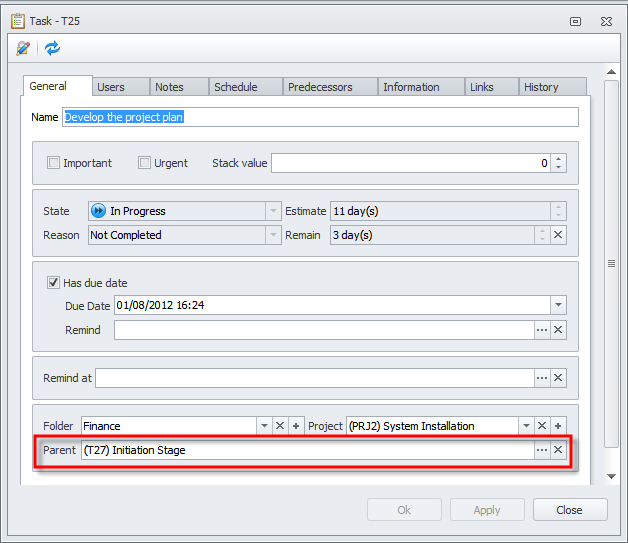When you drag a task to another task, the first task becomes a subtask of the second one.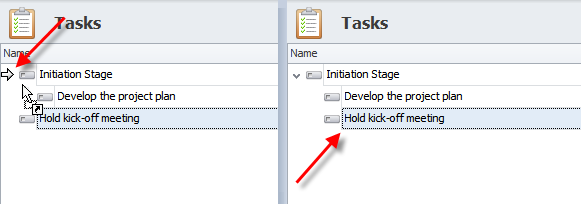Note To create subtasks by drag'n'drop method, click Manual Sorting on the ribbon, in the Extended group.

When you add subtasks to task, some of its properties become dependent on subtasks.

• Task's Estimate and Remain are calculated as the totals of the subtasks’ Estimate and Remain.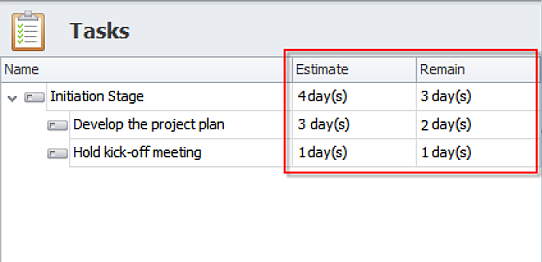• Task's Complete is calculated as average of subtasks' Complete.
• Task's State and Reason are changed automatically depending on subtasks' State and Reason.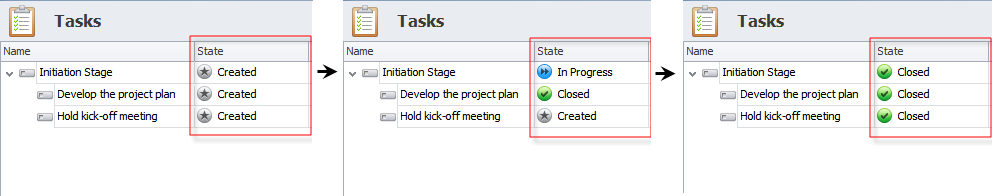• When task's Due Date is earlier than any subtask's Due Date, the font color of task's Due Date becomes red.TipPoint to the task's Due Date to see the details.

Note The latest of subtasks' Due Date is displayed in parentheses.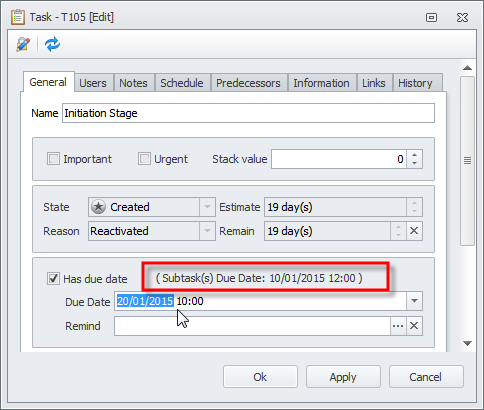Note If you add a subtask to a task with time logs and In Progress state, task's time logs are moved to an additional subtask which is created automatically.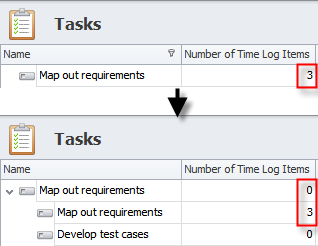If you delete a task that has subtasks, all its subtasks will be deleted.